# RLC Low-Pass Filter Design Tool

This page is a web application that design a RLC low-pass filter. Use this utility to simulate the Transfer Function for filters at a given frequency, damping ratio ζ, Q or values of R, L and C. The response of the filter is displayed on graphs, showing Bode diagram, Nyquist diagram, Impulse response and Step response.

## Calculate the transfer function for RLC low-pass filter with R, L and C values

 Vin(s)→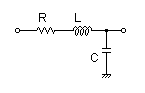→Vout(s)
Transfer function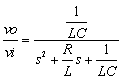R=Ω L=H C=F
p:pico, n:nano, u:micro, k:kilo, M:mega

### Frequency analysis

Bode diagram
Phase  Group delay
Nyquist diagram
Pole, zero
Phase margin
Oscillation analysis

Upper and lower frequency limits:
f1= - f2=[Hz] (frequency limits are optional)

### Transient analysis

Step response
Impulse response
Overshoot
Final value of the step response

Simulation time:
0 - [sec] (optional)

## Calculate the R, L and C values for the RLC low-pass filter at a given frequency and Q factor

 Vin(s)→→Vout(s)
Cut-off frequency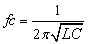Transfer function### Q factor | Damping ratio ζ

Quality factor Q =
Damping ratio ζ =
 fc= Hz L = H C = F
Give two values from three parameters of f0 ,L, C.

Select Capacitor Sequence:
Select Resistor Sequence:
Select Inductance Sequence:

### Frequency analysis

Bode diagram
Phase  Group delay
Nyquist diagram
Pole, zero
Phase margin
Oscillation analysis

Upper and lower frequency limits:
f1= - f2=[Hz] (frequency limits are optional)

### Transient analysis

Step response
Impulse response
Overshoot
Final value of the step response

Simulation time:
0 - [sec] (optional)

## Calculate the transfer function for RLC low-pass filter with R, L and C values

 Vin(s)→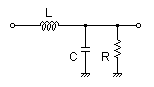→Vout(s)
Transfer function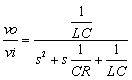R=Ω L=H C=F
p:pico, n:nano, u:micro, k:kilo, M:mega

### Frequency analysis

Bode diagram
Phase  Group delay
Nyquist diagram
Pole, zero
Phase margin
Oscillation analysis

Upper and lower frequency limits:
f1= - f2=[Hz] (frequency limits are optional)

### Transient analysis

Step response
Impulse response
Overshoot
Final value of the step response

Simulation time:
0 - [sec] (optional)

## Calculate the R, L and C values for the RLC low-pass filter at a given frequency and Q factor

 Vin(s)→→Vout(s)
Cut-off frequencyTransfer function### Q factor | Damping ratio ζ

Quality factor Q =
Damping ratio ζ =
 fc= Hz L = H C = F
Give two values from three parameters of f0 ,L, C.

Select Capacitor Sequence:
Select Resistor Sequence:
Select Inductance Sequence:

### Frequency analysis

Bode diagram
Phase  Group delay
Nyquist diagram
Pole, zero
Phase margin
Oscillation analysis

Upper and lower frequency limits:
f1= - f2=[Hz] (frequency limits are optional)

### Transient analysis

Step response
Impulse response
Overshoot
Final value of the step response

Simulation time:
0 - [sec] (optional)